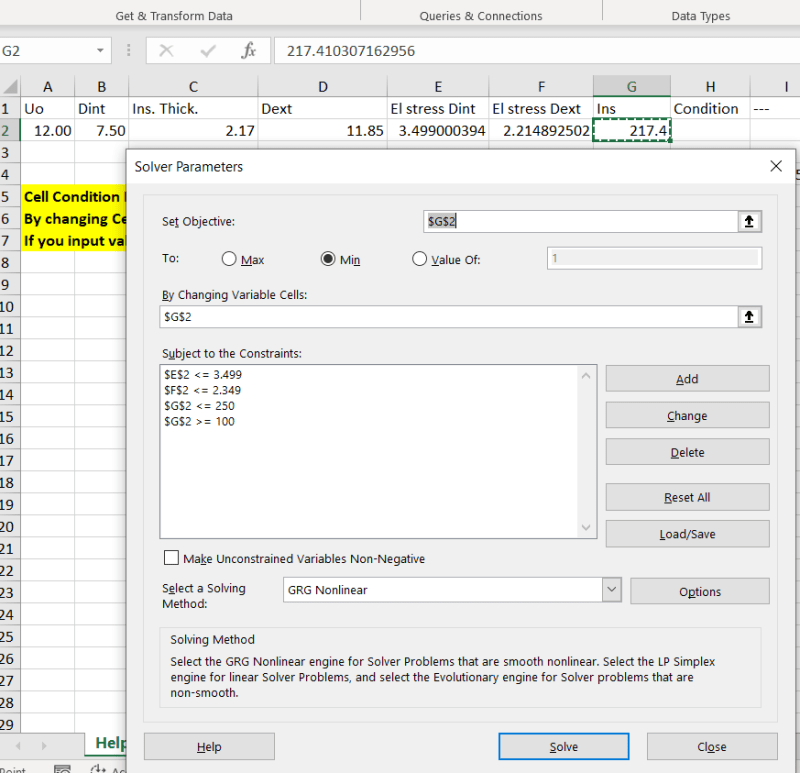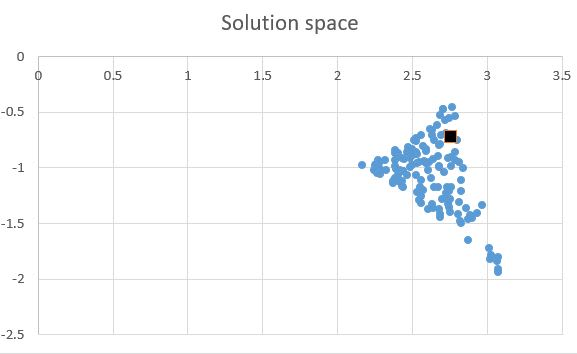×
INTELLIGENT WORK FORUMS
FOR ENGINEERING PROFESSIONALS

Are you an
Engineering professional?
Join Eng-Tips Forums!
• Talk With Other Members
• Be Notified Of Responses
• Keyword Search
Favorite Forums
• Automated Signatures
• Best Of All, It's Free!

*Eng-Tips's functionality depends on members receiving e-mail. By joining you are opting in to receive e-mail.

#### Posting Guidelines

Promoting, selling, recruiting, coursework and thesis posting is forbidden.

# Excel spreadsheet that uses Goal Seek (if possible) to find variable satisfying two conditions

## Excel spreadsheet that uses Goal Seek (if possible) to find variable satisfying two conditions

(OP)

My excel spreadsheet contains a cell with a variable value used in two formulas.
Both of these formulas should have values less than or equal to predetermined numbers, a different number for each formula.
Nevertheless, I must calculate the minimum number satisfying the above formulas, following the logic below:

=IF(OR(AND(E2=3,5;F2<=2,35);AND(F2=2,35;E2<=3,5));VALUE(1);VALUE(0))

where E2, F2 are the cells containing the formulas and 3,5 & 2,35 the predetermined numbers (the variable is contained in a different cell).

The goal seek cannot find an answer unless I have already input the correct value in the cell containing the variable.

What am I missing here?

### RE: Excel spreadsheet that uses Goal Seek (if possible) to find variable satisfying two conditions

When you say "Goal Seek" are you referring to the Data Sovler Add-In?

It looks like you have two fields, El stress Dint and El stress Dext that are both inputs to your Condition (1 if they fall within either of two ranges and 0 if they are outside of them).

Rather than try to point the solver at H2, set your goal to be E2 or F2. If your goal is to find the lowest Ins value that will give you Condition 1, then run two solver scenarios.

Set Objective: $E$2
To: Value of: 3.5
By Changing Variable Cells: $G$2
Subject to the Constraints: $G$2<=1000, $G$2>=1 (or use more realistic values; these are required for the next step to work), and F$2$<=2.35
Select a Solving Method: Evolutionary
(If F$2$<=2.35 is omitted, it gives 217.3 on the first run. With it, you have to solve twice to get the right answer.)

Then run it again for F2=2.35 and E2<=3.5 and use the lower value. Modify procedure to get what you actually need from the data.

If you'll be using this a lot, it would be worthwhile to write a VBA script to run the solver twice with the various settings, compare the values, and provide the desired result(s).

### RE: Excel spreadsheet that uses Goal Seek (if possible) to find variable satisfying two conditions

Getting back to the original solver issue, you need to understand why your cost function crashed and burned. Solvers, such as the one in Excel, depend on being able to find the direction toward the correct solution from the guess value. This is done by looking at the slope of the cost function at the guess value. This generally requires, then, that the cost function has a continuous derivative. Your cost function is a delta function, so there's no slope for the solver to follow.

As a general rule, Boolean tests are bad choices for cost functions, because they're often discontinuous and rarely have continuous derivatives.

TTFN (ta ta for now)
I can do absolutely anything. I'm an expert! https://www.youtube.com/watch?v=BKorP55Aqvg
FAQ731-376: Eng-Tips.com Forum Policies forum1529: Translation Assistance for Engineers Entire Forum list http://www.eng-tips.com/forumlist.cfm

### RE: Excel spreadsheet that uses Goal Seek (if possible) to find variable satisfying two conditions

IRstuff - are you speaking specifically about the GRG Nonlinear solver?

Here's the blurb the solver dialog gives you to guide you in the choice of solver settings:

#### Quote (Excel Solver)

Solving Method Select the GRG Nonlinear engine for Solver Problems that are smooth nonlinear. Select the LP Simplex engine for linear solver Problems, and select the Evolutionary engine for Solver problems that are non-smooth.

So just as IRstuff said, using GRG Nonlinear needs to look at the slope at the initial value. If you don't have that, you can use Evolutionary which creates a "population" of the data, tests it, and then creates a new one from the results, and continues through multiple "generations" to see how the result is "evolving." Once it stops evolving (converges), you get an answer.

Here's a website that explains the solver functions in just enough detail to get the gist: https://www.engineerexcel.com/excel-solver-solving-method-choose/

### RE: Excel spreadsheet that uses Goal Seek (if possible) to find variable satisfying two conditions

His cost function, as shown in his OP, uses equality tests, which automatically makes that function ill-behaved for any solver, since it's only satisfied at one point and is zero elsewhere.

That's not to mention the ROUND functions, which add another layer of discontinuities

TTFN (ta ta for now)
I can do absolutely anything. I'm an expert! https://www.youtube.com/watch?v=BKorP55Aqvg
FAQ731-376: Eng-Tips.com Forum Policies forum1529: Translation Assistance for Engineers Entire Forum list http://www.eng-tips.com/forumlist.cfm

### RE: Excel spreadsheet that uses Goal Seek (if possible) to find variable satisfying two conditions

The spreadsheet linked in the OP is set up to use the Goal Seek function rather than Solver, so the answer to the original question is that Goal Seek is the wrong tool for the problem. Goal Seek finds a solution to a continuous equation with a single variable.

Solver can be set up to minimise a function, subject to specified constraints.
Removing the Round functions from the stress equations, Solver finds a minimum Ins value of 217.4 to satisfy both stress constraints. It will work with any of the solvers, but the evolutionary solver is very slow, and the GRG Nonlinear solver is near instantaneous.

Note that the solver add-in is not enabled by default. Go to File-Options-Add-ins Manage Excel Add-ins if it does not appear at the right hand end of the Data Ribbon.Doug Jenkins
Interactive Design Services
http://newtonexcelbach.wordpress.com/

### RE: Excel spreadsheet that uses Goal Seek (if possible) to find variable satisfying two conditions

One effective method for finding simple optima is to use a Monte Carlo, that is, just random sets of inputs. Engineering judgement can then be used to find the global optimum.

here's the solution space for a 2 factor problem, the black square is a feasible solution using production parts, not quite as good as the optimum at(2.8,-.45) but not bad. This was all done in excel.Cheers

Greg Locock

New here? Try reading these, they might help FAQ731-376: Eng-Tips.com Forum Policies http://eng-tips.com/market.cfm?

### RE: Excel spreadsheet that uses Goal Seek (if possible) to find variable satisfying two conditions

IDS - that's odd. I ran the same thing but the GRG Nonlinear kept coming back with a fault for me. I could only get it with Evolutionary (slow though it is).

### RE: Excel spreadsheet that uses Goal Seek (if possible) to find variable satisfying two conditions

I had another go at this with Goalseek.

I set up two cells (E4 and F4) with the difference between the maximum allowed stresses and the calculated values, then I changed the "condition" formula in H2 to:
=IF(MIN(E4:F4)<0,1,MIN(E4:F4))*1000.

You can then use goalseek to adjust G2 so that H2 is close to zero.

It's a bit slow, and works best if the starting value is greater than the optimum, but it does work. Starting with a value below the optimum it often comes up with a poor approximation. In that case just accept the poor answer and run it again with that as the starting point.

Edit:

That's over complicated. Changing the condition formula to:
=MIN(E4:F4)*1000

is simpler and works better with starting values less than the optimum.

Doug Jenkins
Interactive Design Services
http://newtonexcelbach.wordpress.com/

#### Red Flag This Post

Please let us know here why this post is inappropriate. Reasons such as off-topic, duplicates, flames, illegal, vulgar, or students posting their homework.

#### Red Flag Submitted

Thank you for helping keep Eng-Tips Forums free from inappropriate posts.
The Eng-Tips staff will check this out and take appropriate action.

#### Resources

Low-Volume Rapid Injection Molding With 3D Printed Molds
Learn methods and guidelines for using stereolithography (SLA) 3D printed molds in the injection molding process to lower costs and lead time. Discover how this hybrid manufacturing process enables on-demand mold fabrication to quickly produce small batches of thermoplastic parts. Download Now
Examine how the principles of DfAM upend many of the long-standing rules around manufacturability - allowing engineers and designers to place a partâ€™s function at the center of their design considerations. Download Now
Industry Perspective: Education and Metal 3D Printing
Metal 3D printing has rapidly emerged as a key technology in modern design and manufacturing, so itâ€™s critical educational institutions include it in their curricula to avoid leaving students at a disadvantage as they enter the workforce. Download Now

Close Box

# Join Eng-Tips® Today!

Join your peers on the Internet's largest technical engineering professional community.
It's easy to join and it's free.

Here's Why Members Love Eng-Tips Forums:

•Talk To Other Members
• Notification Of Responses To Questions
• Favorite Forums One Click Access
• Keyword Search Of All Posts, And More...

Register now while it's still free!